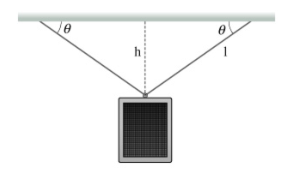# Problem: A loudspeaker of mass 19.0 kg is suspended a distance of h = 2.40 m below the ceiling by two cables that make equal angles with the ceiling. Each cable has a length of I = 3.30 m. (Figure 1)What is the tension T in each of the cables? Use 9.80 m/s2 for the magnitude of the acceleration due to gravity.

###### FREE Expert Solution

Newton's second law:

$\overline{){\mathbf{\Sigma }}{\mathbf{F}}{\mathbf{=}}{\mathbf{m}}{\mathbf{a}}}$

We'll use trigonometry and Newton's second law to solve this problem.

From the diagram:

sinθ = h/l

θ = sin-1 (h/l) = sin-1 (2.40/3.30) = 46.7°

98% (127 ratings)###### Problem Details

A loudspeaker of mass 19.0 kg is suspended a distance of h = 2.40 m below the ceiling by two cables that make equal angles with the ceiling. Each cable has a length of I = 3.30 m. (Figure 1)What is the tension T in each of the cables? Use 9.80 m/s2 for the magnitude of the acceleration due to gravity.